Symmetry quiz #1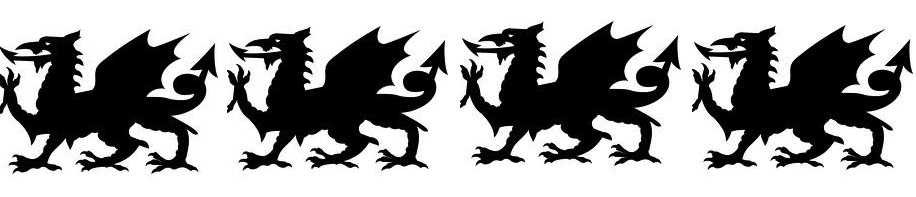1. Name the above band or line symmetry type *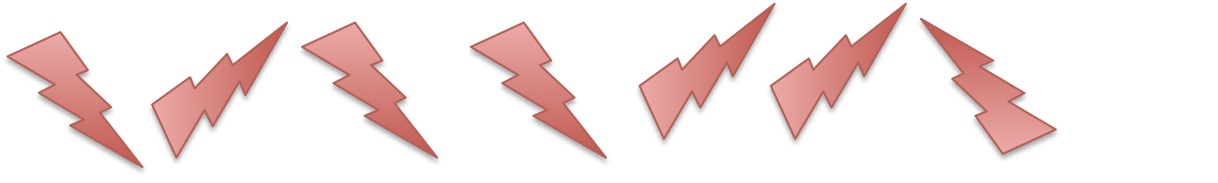2. Name the above band or line symmetry type *3. Which symmetry type is this in the space above? *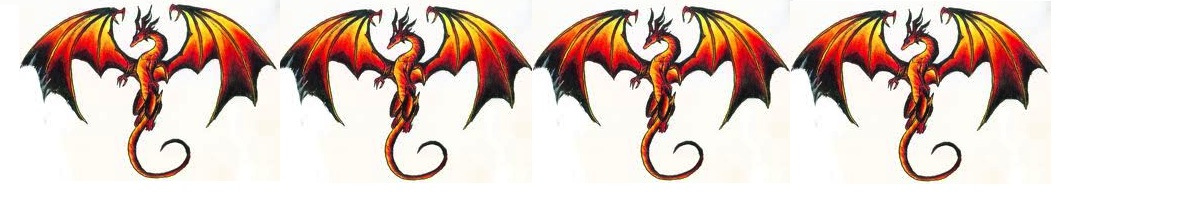4. Name the above symmetry *5. Name the above band or line symmetry type *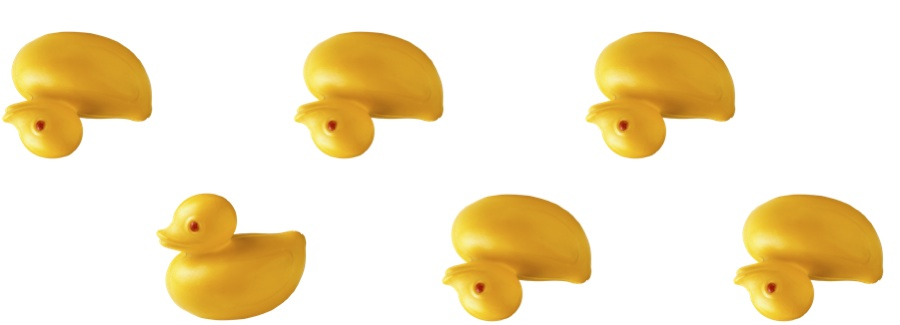6. Name the above band or line symmetry type *7. Name the above band or line symmetry type *8. Name the symmetry above *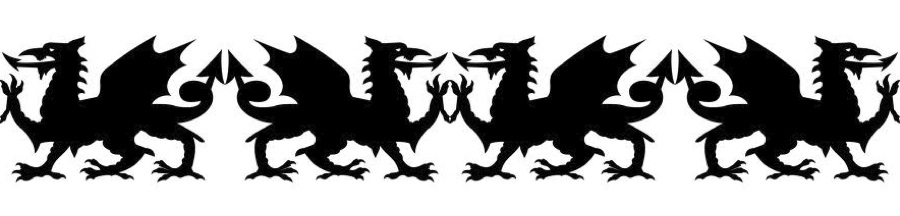9. Name the above band or line symmetry type *10. Name the above band or line symmetry type *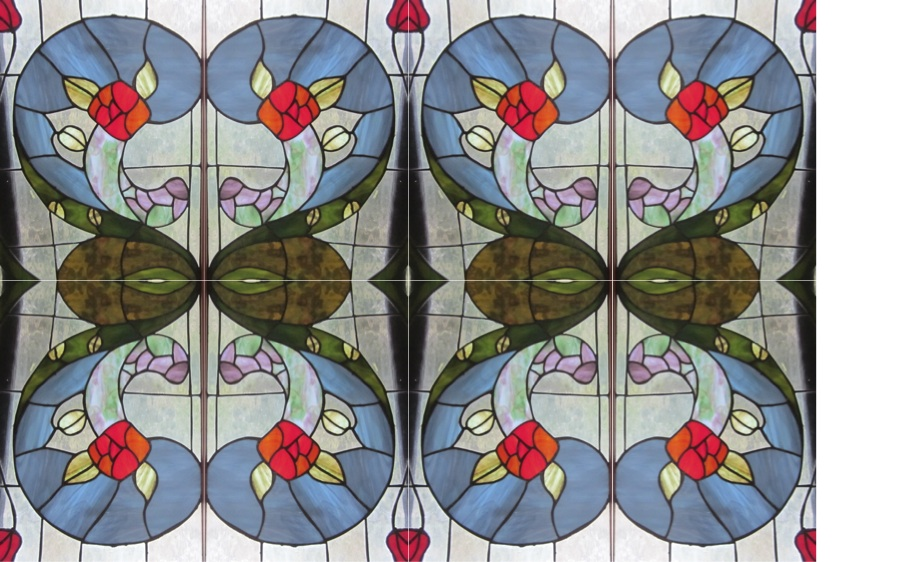11. Name the above band or line symmetry type *